• 2021-06-07 20:32:40

本实验使用环境为Anaconda3 Jupyter，调用Sklearn包，调用keras包,请提前准备好。

## 1.引入一些常见包

主要有keras包、numpy包、metrics包、pandas包等。

import csv
import numpy as np
import time
from sklearn.preprocessing import StandardScaler
from sklearn.model_selection import train_test_split
from sklearn.ensemble import RandomForestClassifier
from sklearn.metrics import accuracy_score
from sklearn.metrics import confusion_matrix
from sklearn.metrics import classification_report
from sklearn.metrics import explained_variance_score
from sklearn import metrics
from sklearn.svm import SVR
import matplotlib.pyplot as plt
from pandas import DataFrame
from pandas import Series
from pandas import concat
from pandas import datetime
from sklearn.metrics import mean_squared_error
from sklearn.preprocessing import MinMaxScaler
from keras.models import Sequential
from keras.layers import Dense
from keras.layers import LSTM
from math import sqrt
from matplotlib import pyplot
import numpy


## 2.引入数据

其中data为全部数据、traffic_feature为特征集、traffic_target目标集

data=[]
traffic_feature=[]
traffic_target=[]

for content in csv_file:
content=list(map(float,content))
if len(content)!=0:
data.append(content)
traffic_feature.append(content[0:4])
traffic_target.append(content[-1])

traffic_feature=np.array(traffic_feature)
traffic_target=np.array(traffic_target)
data=np.array(data)


对数据进行分割，本文使用70%为分割点。


feature_train=traffic_feature[0:int(len(traffic_feature)*0.7)]
feature_test=traffic_feature[int(len(traffic_feature)*0.7):int(len(traffic_feature))]
target_train=traffic_target[0:int(len(traffic_target)*0.7)]
target_test =traffic_target[int(len(traffic_target)*0.7):int(len(traffic_target))]


对后30%的目标值继续分割，分割点仍然为70%，预留做对照。

target_test_last=target_test[int(len(target_test)*0.7):int(len(target_test))]


## 3.数据标准化

使用StandardScaler()方法将特征数据标准化归一化。

scaler = StandardScaler() # 标准化转换
scaler.fit(traffic_feature)  # 训练标准化对象
traffic_feature= scaler.transform(traffic_feature)   # 转换数据集


## 4.使用ELM算法预测

class HiddenLayer:
def __init__(self, x, num):  # x：输入矩阵   num：隐含层神经元个数
row = x.shape
columns = x.shape
rnd = np.random.RandomState(9999)
self.w = rnd.uniform(-1, 1, (columns, num))  #
self.b = np.zeros([row, num], dtype=float)  # 随机设定隐含层神经元阈值，即bi的值
for i in range(num):
rand_b = rnd.uniform(-0.4, 0.4)  # 随机产生-0.4 到 0.4 之间的数
for j in range(row):
self.b[j, i] = rand_b  # 设定输入层与隐含层的连接权值
self.h = self.sigmoid(np.dot(x, self.w) + self.b)  # 计算隐含层输出矩阵H
self.H_ = np.linalg.pinv(self.h)  # 获取H的逆矩阵
# print(self.H_.shape)

# 定义激活函数g(x) ，需为无限可微函数
def sigmoid(self, x):
print(x)
return 1.0 / (1 + np.exp(-x))

'''  若进行分析的训练数据为回归问题，则使用该方式 ，计算隐含层输出权值，即β '''

def regressor_train(self, T):
C = 2
I = len(T)
sub_former = np.dot(np.transpose(self.h), self.h) + I / C
all_m = np.dot(np.linalg.pinv(sub_former), np.transpose(self.h))
T = T.reshape(-1, 1)
self.beta = np.dot(all_m, T)
return self.beta

"""
计算隐含层输出权值，即β
"""

def classifisor_train(self, T):
en_one = OneHotEncoder()
# print(T)
T = en_one.fit_transform(T.reshape(-1, 1)).toarray()  # 独热编码之后一定要用toarray()转换成正常的数组
# print(T)
C = 3
I = len(T)
sub_former = np.dot(np.transpose(self.h), self.h) + I / C
all_m = np.dot(np.linalg.pinv(sub_former), np.transpose(self.h))
self.beta = np.dot(all_m, T)
return self.beta

def regressor_test(self, test_x):
b_row = test_x.shape
h = self.sigmoid(np.dot(test_x, self.w) + self.b[:b_row, :])
result = np.dot(h, self.beta)
return result

def classifisor_test(self, test_x):
b_row = test_x.shape
h = self.sigmoid(np.dot(test_x, self.w) + self.b[:b_row, :])
result = np.dot(h, self.beta)
result = [item.tolist().index(max(item.tolist())) for item in result]
return result


跑起来～
设置神经元个数为8，可以自行调优。

import matplotlib.pyplot as plt
from sklearn.metrics import explained_variance_score
a = HiddenLayer(feature_train,8)
a.regressor_train(target_train)
result = a.regressor_test(feature_test)
plt.plot(result)#测试数组
plt.plot(target_test)#测试数组
plt.legend(['ELM','TRUE'])
fig = plt.gcf()
fig.set_size_inches(18.5, 10.5)
plt.title("ELM")  # 标题
plt.show()
print("EVS:",explained_variance_score(target_test,result))


结果如下：
EVS等于0.8705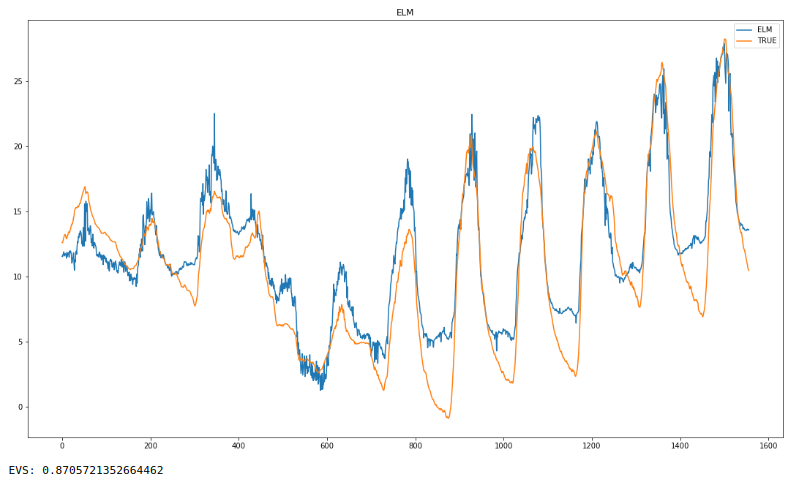## 5.使用真实值减预测值，获的ELM的残差

a=[]#真实值
for i in target_test:
a.append(i)
b=[]#预测值
for i in result:
b.append(i)

c=[]#残差值
num=[]
for inx,i in enumerate(a):
c.append(b[inx]-i)
num.append(inx)
plt.plot(c)#残差
fig = plt.gcf()
fig.set_size_inches(18.5,5)
plt.xlim(0,1560)
plt.title("Residual Signal")  # 标题
plt.show()


残差值变化如下：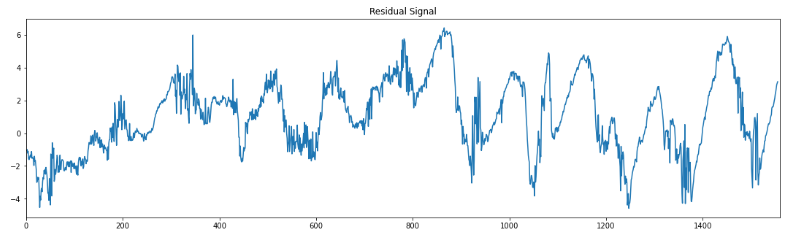将预测的后30%截取，预留做对照。

result_last=b[int(len(b)*0.7):int(len(b))]


## 6.对残差值使用长短记忆神经网络（LSTM）预测

使用残差值的前70%作为测试集，使用后30%作为验证集。

train, test =c[0:int(len(c)*0.7)], c[int(len(c)*0.7):int(len(c))]

def timeseries_to_supervised(data, lag=1):
df = DataFrame(data)
columns = [df.shift(i) for i in range(1, lag+1)]
columns.append(df)
df = concat(columns, axis=1)
df.fillna(0, inplace=True)
return df
def fit_lstm(train, batch_size, nb_epoch, neurons):
X, y = train[:, 0:-1], train[:, -1]
X = X.reshape(X.shape, 1, X.shape)
model = Sequential()
for i in range(nb_epoch):
model.fit(X, y, epochs=1, batch_size=batch_size, verbose=0, shuffle=False)
model.reset_states()
return model
# make a one-step forecast
def forecast_lstm(model, batch_size, X):
X = X.reshape(1, 1, len(X))
yhat = model.predict(X, batch_size=batch_size)
return yhat[0,0]

c2d=[]
for i in c:
c2d.append([i,i])
scaler = StandardScaler() # 标准化转换
scaler.fit(c2d)  # 训练标准化对象
supervised= scaler.transform(c2d)   # 转换数据集
c1d=[]
for j in supervised:
c1d.append(j)
supervised = timeseries_to_supervised(c1d, 1)
train_scaled, test_scaled  =supervised[0:int(len(supervised)*0.7)], supervised[int(len(supervised)*0.7):int(len(supervised))]

train_scaled=np.array(train_scaled)
test_scaled=np.array(test_scaled)

print("开始")
# fit the model
lstm_model = fit_lstm(train_scaled, 1, 30, 4)
# forecast the entire training dataset to build up state for forecasting
train_reshaped = train_scaled[:, 0].reshape(len(train_scaled), 1, 1)
lstm_model.predict(train_reshaped, batch_size=1)

# walk-forward validation on the test data
predictions = list()
for i in range(len(test_scaled)):
# make one-step forecast
X, y = test_scaled[i, 0:-1], test_scaled[i, -1]
yhat = forecast_lstm(lstm_model, 1, X)
# store forecast
predictions.append(yhat)
print("结束")


经过数据格式改变后，残差预测效果如下。

predictions2d=[]
for i in predictions:
predictions2d.append([i,i])
predictions2d
predictions2d=scaler.inverse_transform(predictions2d)
predictions1d=[]
for j in predictions2d:
predictions1d.append(j)

# report performance
rmse = sqrt(mean_squared_error(test, predictions1d))
print('RMSE: %.3f' % rmse)
# line plot of observed vs predicted
fig = pyplot.gcf()
fig.set_size_inches(18.5, 10.5)
pyplot.plot(test)
pyplot.plot(predictions1d)
pyplot.show()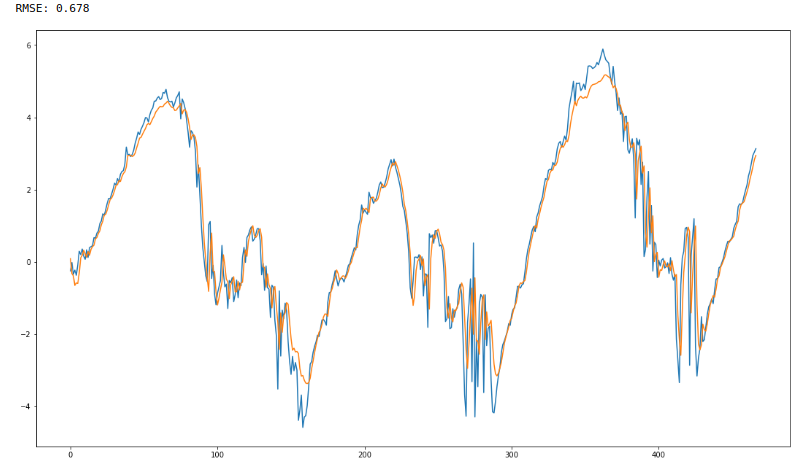## 7.ELM与ELM-LSTM预测结果进行对比

ELM：

print("mse：",metrics.mean_squared_error(target_test_last,result_last))
print("R2:",metrics.r2_score(target_test_last,result_last))ELM-LSTM：

test1=np.array(test)
predictions1d1=np.array(predictions1d)
result1=result_last-test1+predictions1d1
print("mse：",metrics.mean_squared_error(target_test_last,result1))
print("R2:",metrics.r2_score(target_test_last,result1))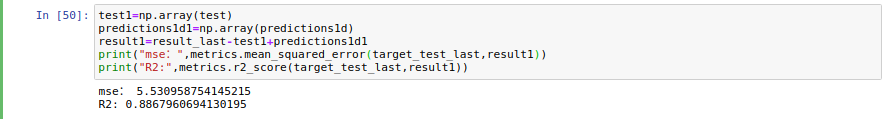COMPARE：

x=range(1089,1556,1)
plt.plot(x,target_test_last,marker=',')
plt.plot(x,result_last,marker='+')
plt.plot(x,result1,marker='x')
plt.legend(['True','ELM','ELM_LSTM'])
fig = plt.gcf()
fig.set_size_inches(18.5, 10.5)
plt.title("COMPARE")  # 标题
plt.show()


结果如下：
由上可知ELM-LSTM比ELM的R方要高、MSE要低。且下图显示ELM-LSTM比ELM更贴近真实值。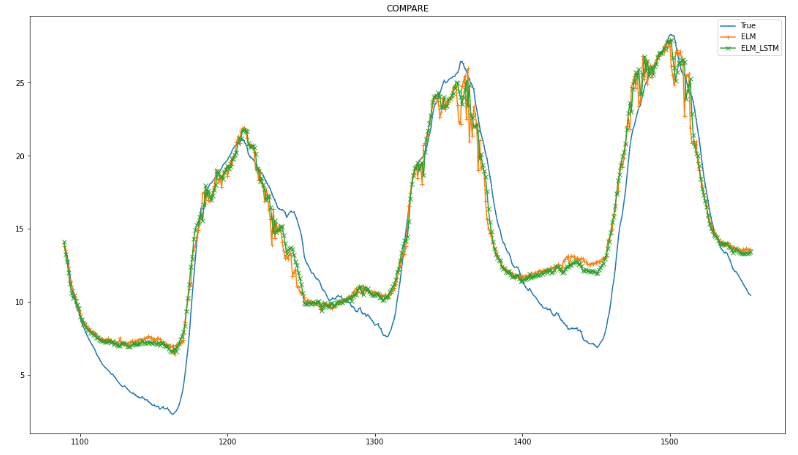## 提供思路

由于数据并非我本人所有，所以我不能提供给大家。数据格式如下：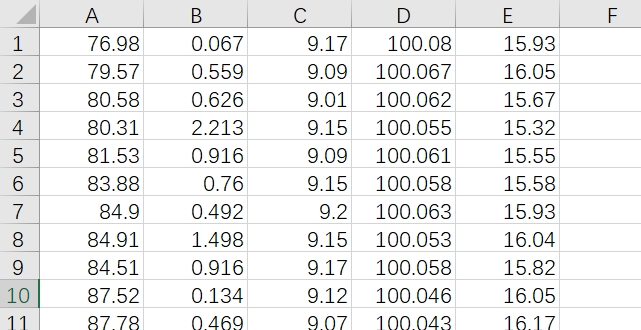更多相关内容
• 基于长短记忆神经网络的短期光伏发电预测技术研究.pdf
• 长短记忆神经网络在流行性感冒暴发预测中的应用.pdf
• 联合卷积与长短记忆神经网络的桥梁结构损伤识别研究.pdf
• 网络游戏-引导滤波长短记忆神经网络高光谱遥感图像的分类方法.zip
• 长短记忆网络(Long Short Term Memory Network, LSTM)，它有效地解决了原始循环神经网络(RNN)的缺陷，在语音识别、图片描述、自然语言处理等许多领域中成功应用。本文讲解由三个Gate（input、forget、output）和一...
• ## 长短时记忆神经网络（LSTM）

万次阅读 多人点赞 2020-09-24 20:27:36
下面为翻译文章，会稍有增删： ...循环神经网络 人类不会每秒都从头开始思考。 阅读本文时，您会根据对先前单词的理解来理解每个单词。 您不会丢掉一切，重新从头开始思考。 传统的神经网络无法做到这一

下面为翻译文章，会稍有增删：

##### 循环神经网络

人类不会每秒都从头开始思考。 阅读本文时，您会根据对先前单词的理解来理解每个单词。 您不会丢掉一切，重新从头开始思考。 传统的神经网络无法做到这一点，这似乎是一个重大缺陷。 例如，假设您想对电影中每个点发生的事件进行分类。 尚不清楚传统的神经网络如何利用电影中先前事件的推理来告知后期事件。循环神经网络解决了这个问题。 它是具有循环的网络，可以使信息持久存在。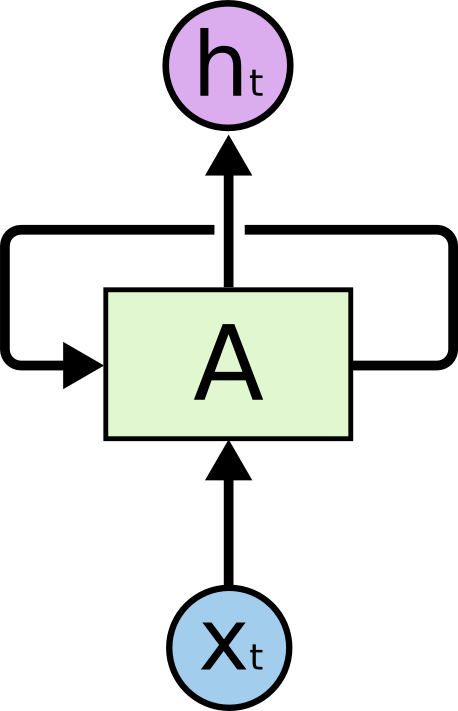图1 循环神经网络

上面的循环神经网络，以 x t x_t 作为网络的输入，然后输出 h t h_t ，循环使得信息可以从当前步流动到下一步。

这些循环使这个神经网络显得有些神秘。 但是，如果您再想一想，就会发现它们与普通的神经网络并没有什么不同。 循环神经网络可以看作是同一网络的多个副本，每个副本都将消息传递给后继者。 考虑一下如果展开循环会发生什么：图2 展开的循环神经网络

这种类似链的性质表明，循环神经网络与序列和列表密切相关。 它们是用于此类数据的神经网络的自然架构。

在过去的几年中，将RNN应用到各种问题上已经取得了令人难以置信的成功：语音识别，语言建模，翻译，图像字幕…清单还在继续。 我将在Andrej Karpathy的出色博客文章 “The Unreasonable Effectiveness of Recurrent Neural Networks” 中讨论使用RNN可以实现的惊人成就。 但是它们确实非常惊人。

这些成功的关键是使用“ LSTM”，这是一种非常特殊的循环神经网络，在许多任务上都能比标准版本好得多。 几乎所有令人兴奋的结果都是利用循环神经网络实现的。 本文将探讨的是这些LSTM

##### 长时依赖问题

RNNs有吸引力的一个地方是它可以将先前的信息连接到当前的任务上，例如使用先前的视频帧可能会有助于对当前帧的理解。如果RNNs可以做到这一点，将变得十分有用，但是可以做到吗？

有时，我们只需要查看最近的信息即可执行当前任务。 例如，考虑一种语言模型，该模型试图根据先前的单词预测下一个单词。 如果我们试图预测 “the clouds are in the sky” 的最后一个词，那么我们不需要任何进一步的上下文——很明显，下一个词将是“sky”。 在这种情况下，相关信息与所需信息之间的距离很小，RNN可以学习使用过去的信息。图3

但是这里也存在一些需要更多上下文信息的情况。想一下如果我们想要预测最后一个单词 “I grew up in France… I speak fluent French.” 从最近的信息来看，要预测的单词应该是一种语言的名字，但是要确定是那种语言，我们需要更前面单词 “France” 的上下文信息。这说明相关信息与当前任务的距离可能非常远。图4

从理论上来说，RNNs完全有能力处理这样的长时依赖，人类可以仔细的选择参数以解决这类简单问题。不幸的是，在实践中，RNNs看起来并没有能力处理他们。Hochreiter（1991）[German]Bengio, et al. (1994)等人对此问题进行了深入探讨，发现了处理这类问题变困难的根本原因。

谢天谢地，LSTMs没有这个问题。

##### LSTM网络

长短时记忆神经网络（Long Short Term networks），通常叫做LSTMs，是一种能够捕捉长时依赖的特殊循环神经网络。它们由Hochreiter & Schmidhuber (1997) 提出，然后被许多人在后来的工作中进行精炼和推广。他们的工作在各种问题上表现都非常好，现在也被广泛使用。

LSTMs是专门设计用来避免长时依赖问题的。长时间的记住信息已经成为了它的默认行为，不需要专门去学习。

所有的LSTMs都有重复神经网络模块的链式形式。在标准的RNNs中，这个重复的模块可以是非常简单的结构，例如单个tanh层。图5 包含单个tanh层的标准RNN重复模块

LSTMs同样也有类似的链式结构，但是重复的模块有不同的结构。不是只有一个神经网络层，而是四个，以一种非常特殊的方式进行交互。图6 包含四个交互层的LSTM重复模块

不需要担心在模型中计算的细节。我们之后将一步步浏览整个LSTM网络。现在先来熟悉一下将要使用到的符号表示。图7 网络中的符号含义
1. 神经网络层（用于学习）
2. 逐点操作（逐点相乘、逐点相加）
3. 向量转移（向量沿箭头方向移动）
4. 连接（将两个向量连接在一起）
5. 复制（将向量复制为两份）
##### LSTMs背后的核心思想

LSTMs的关键是细胞状态（cell state），水平的线贯穿图的顶部。细胞状态就像一个传送带，它沿整个链条一直沿直线延伸，只有一些较小的线性相互作用。 信息不加改变地流动非常容易。图8

LSTM有能力向细胞状态添加或者移除信息，这些操作由门（gate）结构来精细调控。门结构是一种让信息有选择通过的方式。他们由一个sigmoid神经网络层和一个点乘操作构成。图9

sigmoid层输出的数据在0-1之间，表示每一个组件应该通过多少信息。如果为0则表示任何信息都无法通过，如果为1则表示所有信息都可以通过。

一个LSTM有三个这样的门来保护和控制细胞状态。

##### 一步步看LSTM

LSTM的第一个步骤是决定需要从细胞状态中丢弃哪些信息。这个是由一个sigmoid层也叫做遗忘门（forget gate）来决定的。它输入 h t − 1 h_{t-1} x t x_t ，然后经过sigmoid层后，得到0-1之间的输出 f t f_t 。之后跟细胞状态 C t − 1 C_{t-1} 逐点相乘。如果 f t f_t 对应的值为0，则丢弃对应的信息，反之，则保留对应的信息。图10

解释一下：

C t − 1 C_{t-1} 是上一时刻的细胞状态

h t − 1 h_{t-1} 是上一时刻的模块输出

σ \sigma 代表sigmoid层，每一个数据都需要经过sigmoid函数

f t f_t 代表遗忘门的输出

W f W_f 代表权重矩阵

b f b_f 代表偏置

[ h t − 1 , x t ] [h_{t-1},x_t] 代表将两个矩阵拼接在一起

LSTM的下一个步骤是要决定将哪些信息存储在细胞状态中，这包含两个部分：

1.首先有一个sigmoid层叫做“输入门层”，它决定我们将更新哪些信息。

2.下一步，一个tanh层创建候选值的向量， C ~ t \tilde{C}_t ,决定哪些信息能够被加到细胞状态中

接下来，我们将结合着两个部分对细胞状态进行更新。图11

现在我们来将细胞状态 C t − 1 C_{t-1} 更新为 C t C_t

我们先用旧的状态 C t − 1 C_{t-1} 对应点乘 f t f_t ，即 C t − 1 ∗ f t C_{t-1} * f_t ,用来丢弃我们已经决定要遗忘的信息，然后再加上 i t ∗ C ~ t i_t * \tilde{C}_t ，最终构成细胞状态 C t C_t 。这是新的候选值，根据我们决定更新每个状态值的大小来缩放。图12

最后我们需要决定我们的输出，输出取决于我们的细胞状态，但是应当是一个过滤的版本。首先，首先我们要运行一个sigmoid层来决定要输出细胞状态的哪些信息。然后将细胞状态通过tanh层然后乘以sigmoid层的输出，最后我们就可以输出我们想要输出的部分。图12
##### 长短时记忆神经网络的变体

我们到目前为止讨论的都是普通的长短时记忆神经网络。但是并不是所有的LSTMs都跟上面的一样。事实上，几乎每一个涉及到LSTMs的文章都使用了一些不同的版本。这些版本的区别很小，但是值得一提。

其中一个受欢迎的LSTM变体，由Gers & Schmidhuber (2000)提出，添加了窥视孔连接，这意味着可以让门层看到细胞状态。图13

上面的图对每一个门都添加了窥视孔，但是很多文章都仅仅给一部分门窥视孔。

另一个变体使用了双输入和输出门。与单独决定要遗忘或者添加一些信息不同，这个网络同时决定这两件事情。当我们要添加一些信息的时候，才会遗忘这个地方的信息；当我们要遗忘更旧信息的时候，才会在这个地方添加新值。这两句话看起来是在表述一个意思，但是仔细品品，又有些不一样的意味。图14

一个有些戏剧性的LSTM变体是门控循环单元（Gate Recurrent Unit，GRU）Cho, et al. (2014)。它结合了遗忘和输入门成为一个单一的“更新门”，它还结合了细胞状态和隐藏状态，并且做了一些其他的改变。最终的模型比标准的LSTM模型更简单，而且越来越受欢迎。图15

这里仅仅只有一些值得注意的LSTM变体。还有许多其他的例如：Depth Gated RNNs by Yao, et al. (2015) 。同样也有完全不同的方法去解决长时依赖，像Clockwork RNNs by Koutnik, et al. (2014)

这些LSTM变体中那个是最好的？差异重要吗？Greff, et al. (2015) 对受欢迎的变体做了一个比较，发现他们都是一样的。Jozefowicz, et al. (2015) 测试了超过一万个RNN结构，发现一些RNN在特定任务上表现要比LSTMs更好。

##### 总结

之前，我提到了人们使用RNN所取得的非凡成就。基本上所有这些都是使用LSTM实现的。对于大多数任务，它们确实工作得更好！

写成一组方程式，LSTM看起来很吓人。希望本文逐步介绍它们，使他们变得更加平易近人。

LSTM是我们可以使用RNN完成的重要一步。很自然地想：还有另外一个大步吗？研究人员普遍认为：“是的！有下一步，Attention！”这个想法是让RNN的每一步都从更大的信息集合中选择信息。例如，如果您使用RNN创建描述图像的标题，则它可能会选择图像的一部分以查看其输出的每个单词。实际上，Xu, et al. (2015) 正是这样做的-如果您想探索Attention，这可能是一个有趣的起点！Attention取得了许多令人振奋的结果，而且似乎还有很多其他的任务……

Attention不仅仅是RNN研究中唯一令人兴奋的话题。例如，Grid LSTMs by Kalchbrenner, et al. (2015) 看起来更具有前途。将RNNs用于生成模型中也十分有趣，例如： Gregor, et al. (2015), Chung, et al. (2015), 以及 Bayer & Osendorfer (2015) 。最近几年对于递归神经网络来说是一个令人振奋的时刻，而即将到来的几年有望如此！

##### 致谢

展开全文LSTM 深度学习 循环神经网络
• 最近看NBA被篮网队所吸引，有进攻有防守而且观赏性很强，所以特此使用深度学习算法来分析一下篮网队赢球的关键因素有哪些，同时也简单预测一下下一场比赛的结果，仅供参考。 数据来源：布鲁克林篮网队20-21赛季数据...

最近看NBA被篮网队所吸引，有进攻有防守而且观赏性很强，所以特此使用算法来分析一下篮网队赢球的关键因素有哪些，同时也简单预测下一场比赛结果，仅供参考。数据来源：布鲁克林篮网队20-21赛季数据
http://slamdunk.sports.sina.com.cn/team/stats?tid=583ec9d6-fb46-11e1-82cb-f4ce4684ea4c

## 1.数据预处理

下载好的数据：共包含：日期 比赛 类型 主客场 赛果 时间 得分 投篮 投篮% 三分 三分% 罚球 罚球% 前场板 后场板 总篮板 助攻 失误 抢断 盖帽 犯规 26列数据。
使用Excel公式将文本转化为数值：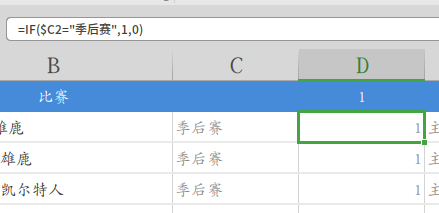其中季后赛=1、常规赛=0、主场=1、客场=0、胜场=1、负场=0等，将全部数据转化为数值类型，删除一些不关心的数据，检索空格，一定要删除空格！一定要删除空格！一定要删除空格！否则一会读CSV时后有你受的。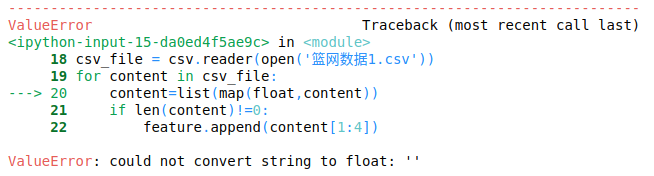（改了半个小时才找到问题…，检索空格替换都不行，最后发现在后面有个“，”…删除就好了。）
最后将Excel转化成CSV文件，将胜负提到第一列。
CSV文件：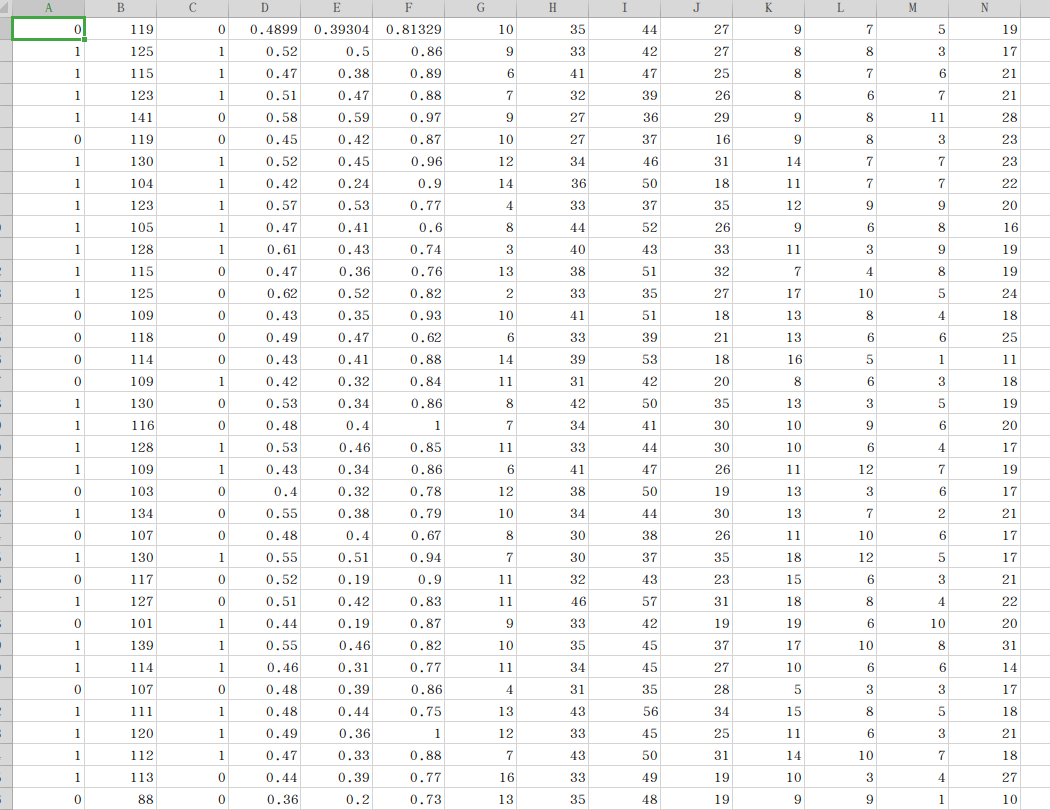## 2.导包

用到了这么多包numpy、xgboost、matplotlib、csv、keras、pandas、metrics等

# plot feature importance using built-in function
from xgboost import XGBClassifier
from xgboost import plot_importance
from matplotlib import pyplot
import numpy as np
import csv
from keras.models import Sequential
from keras.layers import Dense
from keras.layers import LSTM
from sklearn.model_selection import cross_val_score
from sklearn.ensemble import RandomForestClassifier
from sklearn.model_selection import train_test_split
from sklearn.metrics import accuracy_score
from sklearn.metrics import confusion_matrix
from sklearn.metrics import classification_report
from sklearn import metrics
from sklearn.preprocessing import StandardScaler
from pandas import DataFrame
from pandas import Series
from pandas import concat
from pandas import datetime


## 3.导数据

#特征
feature=[]
#目标
target=[]
for content in csv_file:
content=list(map(float,content))
if len(content)!=0:
feature.append(content[1:14])
target.append(content[0:1])
targets=[]
for i in target:
targets.append(i)
targets.reverse()
feature.reverse()
feature=np.array(feature)
targets=np.array(targets)


## 4.使用XGBoost分析影响胜负的关键因素

# fit model no training data
model = XGBClassifier()
model.fit(feature, targets)

# plot feature importance
fig,ax = plt.subplots(figsize=(15,15))
plot_importance(model,
height=0.5,
ax=ax,
max_num_features=64)


结果：
这一堆f什么鬼…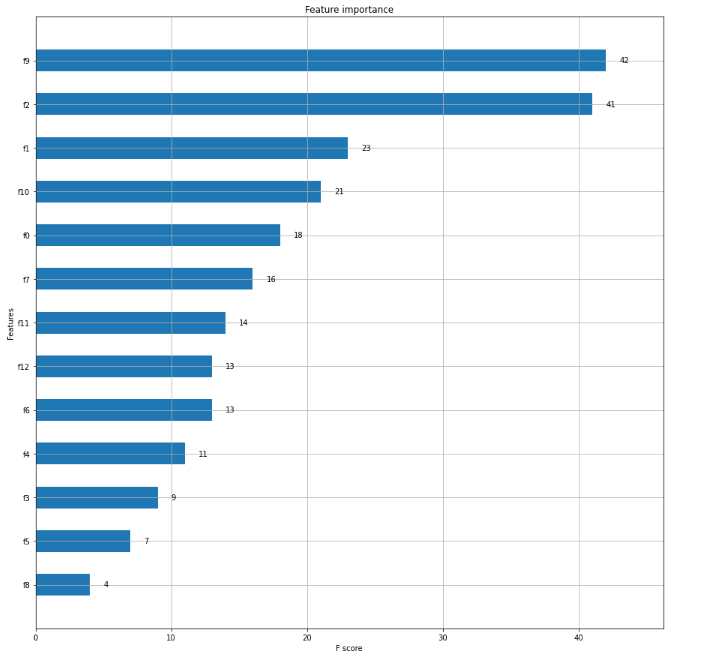把名称对应上：

feature_AllName=['Total','HomeAndAway','FieldGoalPercentage','3-pointShootingPercentage',
'FreeThrowPercentage','TheFrontRebounds','TheBackRebounds','TotalRebounds',
'Assists','Error','Steals','Blocks','foul']
,
feature_name=[feature_AllName,feature_AllName,feature_AllName,feature_AllName
,feature_AllName,feature_AllName,feature_AllName,feature_AllName
,feature_AllName,feature_AllName,feature_AllName,feature_AllName
,feature_AllName]
feature_name.reverse()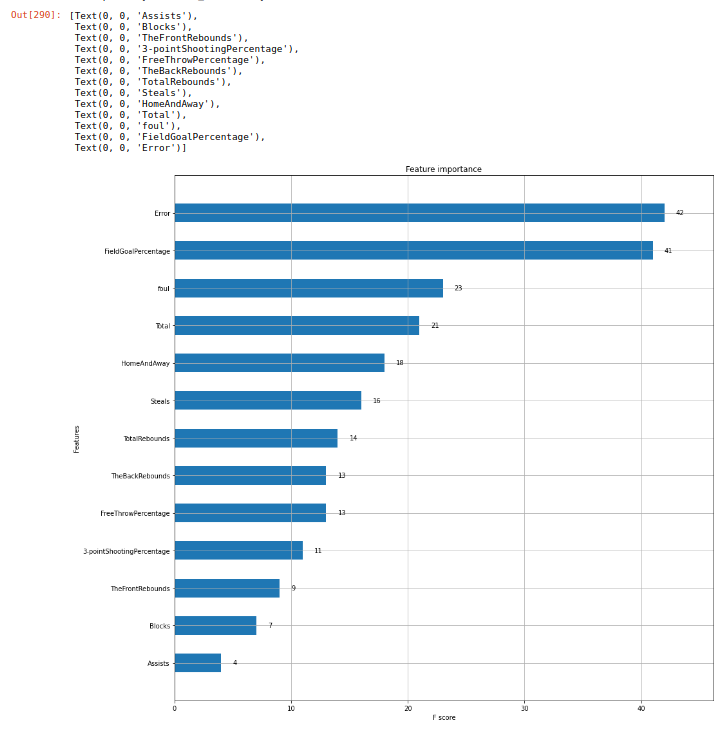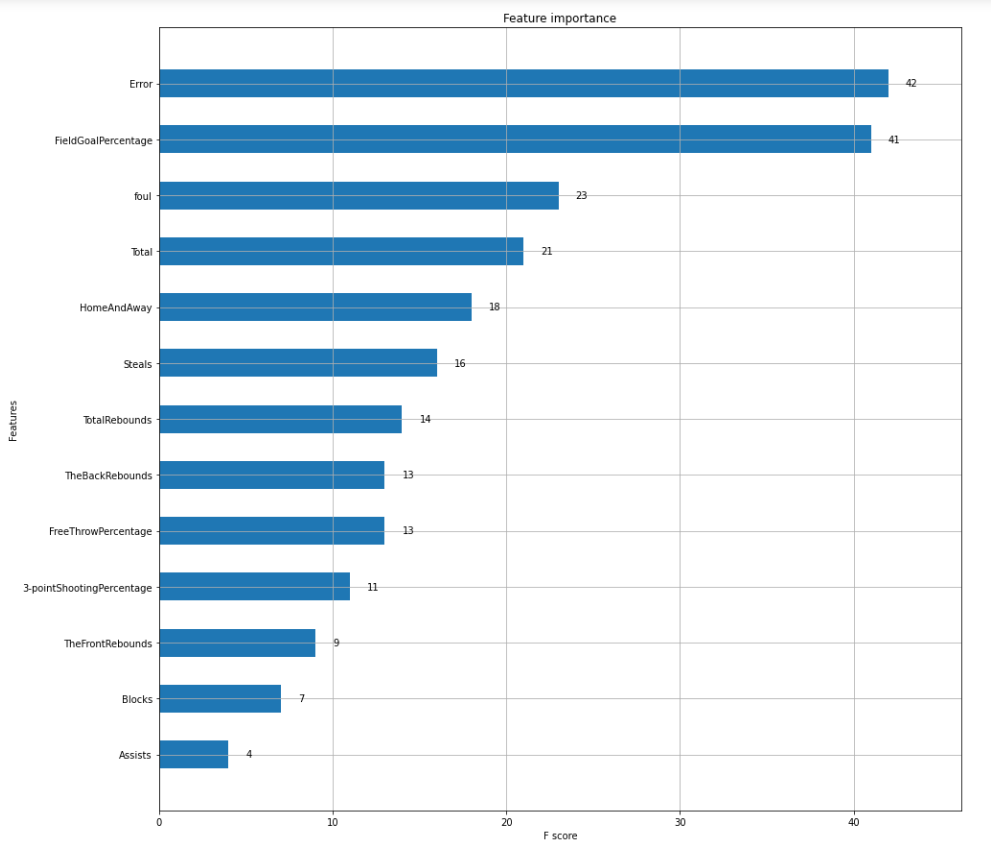没想到啊，对篮网队来说，影响胜负的最关键因素是失误、其次是投篮命中率、接下来是犯规、总分、主客场、抢断…助攻是最不关键的因素。

## 5.使用LSTM对失误、投篮命中率进行单步预测

使用LSTM预测篮网下一场比赛的失误数、投篮命中率。其他因素没有二者关键，直接取平均值了…

重新取出失误数和投篮命中率：

ERROR=[]
FieldGoalPercentage=[]

for content in csv_file:
content=list(map(float,content))
if len(content)!=0:
ERROR.append(content[10:11])
FieldGoalPercentage.append(content[3:4])
Foul.append(content[13:14])
Total.append(content[1:2])
HomeAndAway.append(content[2:3])
ERROR1=[]
for j in ERROR:
ERROR1.append(j)

FieldGoalPercentage1=[]
for j in FieldGoalPercentage:
FieldGoalPercentage1.append(j)


预测失误数：
结果等于：9（8.699947）

train, test =ERROR1[0:int(len(ERROR1)*0.7)],targets[int(len(ERROR1)*0.7):int(len(ERROR1))]

def timeseries_to_supervised(data, lag=1):
df = DataFrame(data)
columns = [df.shift(i) for i in range(1, lag+1)]
columns.append(df)
df = concat(columns, axis=1)
df.fillna(0, inplace=True)
return df
def fit_lstm(train, batch_size, nb_epoch, neurons):
X, y = train[:, 0:-1], train[:, -1]
X = X.reshape(X.shape, 1, X.shape)
model = Sequential()
for i in range(nb_epoch):
model.fit(X, y, epochs=1, batch_size=batch_size, verbose=0, shuffle=False)
model.reset_states()
return model
# make a one-step forecast
def forecast_lstm(model, batch_size, X):
X = X.reshape(1, 1, len(X))
yhat = model.predict(X, batch_size=batch_size)
return yhat[0,0]

c2d=[]
for i in ERROR1:
c2d.append([i,i])
supervised= c2d  # 转换数据集
c1d=[]
for j in supervised:
c1d.append(j)
supervised = timeseries_to_supervised(c1d, 1)
train_scaled, test_scaled  =supervised[0:int(len(supervised)*0.7)], supervised[int(len(supervised)*0.7):int(len(supervised))]

train_scaled=np.array(train_scaled)
test_scaled=np.array(test_scaled)

print("开始")
# fit the model
lstm_model = fit_lstm(train_scaled, 1, 30, 4)
# forecast the entire training dataset to build up state for forecasting
train_reshaped = train_scaled[:, 0].reshape(len(train_scaled), 1, 1)
lstm_model.predict(train_reshaped, batch_size=1)
# walk-forward validation on the test data
predictions = list()
for i in range(len(test_scaled)):
# make one-step forecast
X, y = test_scaled[i, 0:-1], test_scaled[i, -1]
yhat = forecast_lstm(lstm_model, 1,X)
# store forecast
predictions.append(yhat)
print("结束")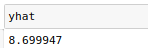预测投篮命中率：
结果等于：0.48989984

train, test =FieldGoalPercentage1[0:int(len(FieldGoalPercentage1)*0.7)],targets[int(len(FieldGoalPercentage1)*0.7):int(len(FieldGoalPercentage1))]

def timeseries_to_supervised(data, lag=1):
df = DataFrame(data)
columns = [df.shift(i) for i in range(1, lag+1)]
columns.append(df)
df = concat(columns, axis=1)
df.fillna(0, inplace=True)
return df
def fit_lstm(train, batch_size, nb_epoch, neurons):
X, y = train[:, 0:-1], train[:, -1]
X = X.reshape(X.shape, 1, X.shape)
model = Sequential()
for i in range(nb_epoch):
model.fit(X, y, epochs=1, batch_size=batch_size, verbose=0, shuffle=False)
model.reset_states()
return model
# make a one-step forecast
def forecast_lstm(model, batch_size, X):
X = X.reshape(1, 1, len(X))
yhat = model.predict(X, batch_size=batch_size)
return yhat[0,0]

c2d=[]
for i in FieldGoalPercentage1:
c2d.append([i,i])
supervised= c2d  # 转换数据集
c1d=[]
for j in supervised:
c1d.append(j)
supervised = timeseries_to_supervised(c1d, 1)
train_scaled, test_scaled  =supervised[0:int(len(supervised)*0.7)], supervised[int(len(supervised)*0.7):int(len(supervised))]

train_scaled=np.array(train_scaled)
test_scaled=np.array(test_scaled)

print("开始")
# fit the model
lstm_model = fit_lstm(train_scaled, 1, 30, 4)
# forecast the entire training dataset to build up state for forecasting
train_reshaped = train_scaled[:, 0].reshape(len(train_scaled), 1, 1)
lstm_model.predict(train_reshaped, batch_size=1)
# walk-forward validation on the test data
predictions = list()
for i in range(len(test_scaled)):
# make one-step forecast
X, y = test_scaled[i, 0:-1], test_scaled[i, -1]
yhat = forecast_lstm(lstm_model, 1,X)
# store forecast
predictions.append(yhat)
print("结束")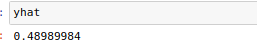## 6.修改原始数据集

预设篮网下一场输了，投篮命中率维0.489、失误9个。其他数值去平均数四舍五入。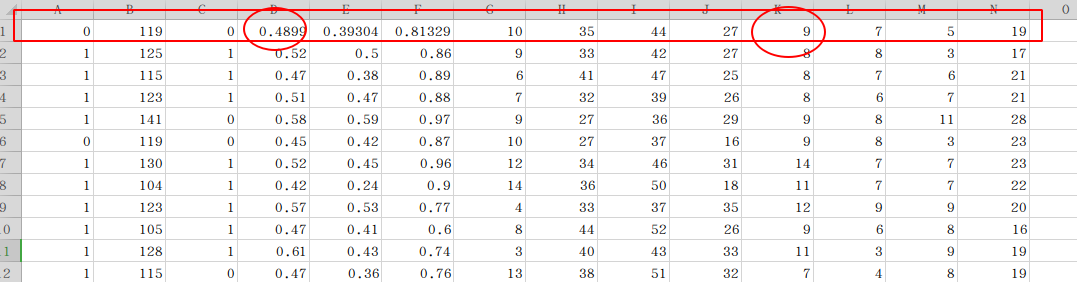重新导入数据集：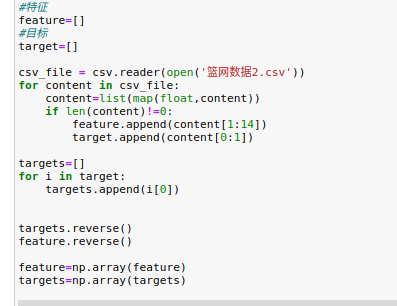## 7.使用随机森林算法（RF）预测篮网队下一场比赛胜负

先显示出篮网队20-21赛季全部胜负场。

plt.plot(targets,marker='s')#测试数组
fig = plt.gcf()
fig.set_size_inches(18.5, 10.5)
plt.title("RF")  # 标题
plt.xlabel('Time')
plt.ylabel('Value')
plt.show()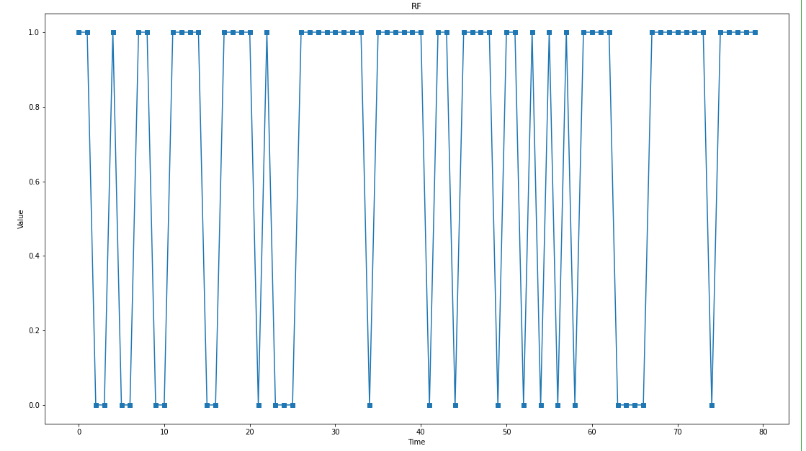使用RF算法：
设置seed为5 防止每次预测效果不同。可以自行修改。
手动分割训练集和测试集，分割点为0.8。

from numpy.random import seed
seed(5)

feature_train=feature[0:int(len(feature)*0.80)]
target_train=targets[0:int(len(targets)*0.80)]
feature_test=feature[int(len(feature)*0.80):int(len(feature))]
target_test=targets[int(len(targets)*0.80):int(len(targets))]
# clf = RandomForestClassifier(criterion='entropy')
clf = RandomForestClassifier()
clf.fit(feature_train,target_train)
predict_results=clf.predict(feature_test)

plt.plot(target_test,marker='s')#测试数组
plt.plot(predict_results,marker='o')#测试数组
plt.legend(['True','RF'])
fig = plt.gcf()
fig.set_size_inches(18.5, 10.5)
plt.title("RF")  # 标题
plt.xlabel('Time')
plt.ylabel('Value')
plt.show()

print(classification_report(target_test, predict_results))


结果如下：
最后一场是随便设置的输球，但RF预测是赢球。
除去最后一场外，其他15场预测对了12场，准确率为80%。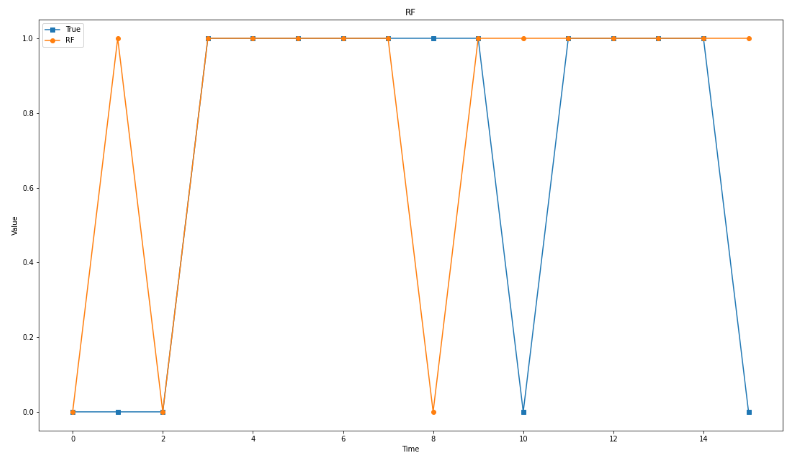进一步求精度：
print(classification_report(target_test, predict_results))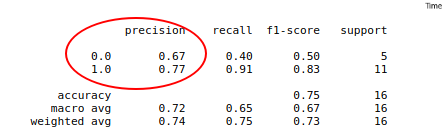可知，预测赢球准确率为77%，预测输球准确率为67%，而RF预测下场赢球，故下一场赢球的可能性为77%！！

以上实验仅供学习参考，真实情况远比数据所描述的情况复杂的多，球员受伤、拉肚子、打架、心情不好、某某球员爆发、裁判原因等情况很多，所以结果仅供娱乐，但是我还是很看好篮网赢球的。

实验中LSTM可以对全部特征都进行预测，也可以可以调参，如果使用优化算法调优相信模型精度还会进一步提高，以此提高可信度。
实验中RF算法也可以调参提高精度，但由于本实验未考虑对手球队，所以对双方球队都进行胜负预测，再对比可能比单方预测的结果更可靠一些，比如预测雄鹿下一场赢球可能性90%，那即使篮网赢球可能性是77%，那么也是篮网输球可能性较大。

算法可供学习，结果仅供参考。

展开全文深度学习
• 人工智能之长短记忆神经网络(LSTM)前言：人工智能机器学习有关算法内容，请参见公众号“科技优化生活”之前相关文章。人工智能之机器学习主要有三大类:1)分类;2)回归;3)聚类。今天我们重点探讨一下长短记忆神经...

人工智能之长短时记忆神经网络(LSTM)

前言：人工智能机器学习有关算法内容，请参见公众号“科技优化生活”之前相关文章。人工智能之机器学习主要有三大类:1)分类;2)回归;3)聚类。今天我们重点探讨一下长短时记忆神经网络(LSTM)算法。 ^_^

通过上一篇文章[人工智能之循环神经网络(RNN)] 介绍，我们知道，RNN是一类功能强大的人工神经网络算法，RNN一个重要的优点在于，其能够在输入和输出序列之间的映射过程中利用上下文相关信息。但是RNN存在着梯度消失或梯度爆炸等问题。因此，为了解决上述问题，长短时记忆神经网络(LSTM)诞生了。

长短期记忆神经网络LSTM是一种特殊的RNN，能够学习长期依赖关系。由Hochreiter和Schmidhuber (1997)提出，在后期工作中又由许多人进行了调整和普及(除了原始作者之外，许多人为现代LSTM做出了贡献，不完全统计：Felix Gers(目前在谷歌的DeepMind任职)， Fred Cummins， Santiago Fernandez， Felix Gers(发明了LSTM遗忘门)，Justin Bayer(自动演化)， Daan Wierstra， Julian Togelius， Faustian Gomez， Matteo Gagliolo 和 Alex Graves)。LSTM在大量问题上效果异常出色，现在正在广泛使用。

LTSM概念:

长短时记忆神经网络LSTM(Long Short–Term Memory)是一种时间递归神经网络，适合于处理和预测时间序列中间隔和延迟相对较长的重要事件。

与其说长短时记忆神经网络LSTM是一种循环神经网络，倒不如说是一个加强版的组件被放在了循环神经网络中。具体地说，就是把循环神经网络中隐含层的小圆圈换成长短时记忆的模块，如下图所示。LTSM本质:

LSTM引入自循环的巧妙构思，以产生梯度长时间持续流动的路径是初始LSTM模型的核心贡献。其中一个关键扩展是使自循环的权重视上下文而定，而不是固定的。门控此自循环(由另一个隐藏单元控制)的权重，累积的时间尺度可以动态地改变。

LSTM循环网络除了外部的RNN循环外，还具有内部的LSTM细胞循环(自环)。

LSTM 通过刻意的设计来避免长期依赖问题。记住长期的信息在实践中是 LSTM 的默认行为，而非需要付出很大代价才能获得的能力。LSTM原理:

LSTM区别于RNN的地方，主要就在于它在算法中加入了一个判断信息有用与否的“处理器”，这个处理器作用的结构被称为cell。

一个cell当中被放置了三扇门，分别叫做输入门、遗忘门和输出门。一个信息进入LSTM的网络当中，可以根据规则来判断是否有用。只有符合算法认证的信息才会留下，不符的信息则通过遗忘门被遗忘。说起来无非就是一进二出的工作原理，却可以在反复运算下解决神经网络中长期存在的大问题。目前已经证明，LSTM是解决长序依赖问题的有效技术，并且这种技术的普适性非常高，导致带来的可能性变化非常多。各研究者根据LSTM纷纷提出了自己的变量版本，这就让LSTM可以处理千变万化的垂直问题。

LSTM深度剖析:

LSTM 有通过精心设计的称作为“门”的结构来去除或者增加信息到细胞状态的能力。门是一种让信息选择式通过的方法。其包含一个 sigmoid 神经网络层和一个 pointwise 乘法操作。Sigmoid 层输出 0 到 1 之间的数值，描述每个部分有多少量可以通过。0 代表“不许任何量通过”，1 就指“允许任意量通过”！

LSTM 拥有三个门(输入门，遗忘门，输出门)，来保护和控制细胞状态。

标准LSTM:

1)决定丢弃信息：2)确定更新的信息:3)更新细胞状态：4)输出信息:LSTM的变体:

1)peephole 连接：2)coupled 忘记门和输入门：3) GRU(Gated Recurrent Unit)：LSTM应用场景：

LSTM已经在科技领域有了多种应用。基于LSTM的系统可以学习翻译语言、控制机器人、图像分析、文档摘要、语音识别、图像识别、手写识别、控制聊天机器人、预测疾病、点击率和股票、合成音乐等任务。2015 年谷歌通过基于CTC 训练的 LSTM 程序大幅提升了安卓手机和其他设备中语音识别的能力。百度也使用了 CTC；苹果的 iPhone 在 QucikType 和 Siri 中使用了LSTM；微软不仅将LSTM 用于语音识别，还将这一技术用于虚拟对话形象生成和编写程序代码等。亚马逊 Alexa 通过双向LSTM在家中与用户交流，而谷歌使用 LSTM 的范围更加广泛，它可以生成图像字幕，自动回复电子邮件，它包含在新的智能助手Allo中，也显著地提高了谷歌翻译的质量。目前，谷歌数据中心的很大一部分计算资源现在都在执行 LSTM 任务。

结语:

长短期记忆网络LSTM是一种时间递归神经网络，适合于处理和预测时间序列中间隔和延迟相对较长的重要事件。LSTM是使用RNN的一个飞跃。LSTM算法在人工智能之机器学习、翻译语言、控制机器人、图像分析、文档摘要、语音识别、图像识别、手写识别、控制聊天机器人、预测疾病、点击率和股票、合成音乐等领域有着广泛应用。

------以往文章推荐------

·        机器学习

·        深度学习

·        人工神经网络

·        决策树

·        随机森林

·        强化学习

·        迁移学习

·        遗传算法

·        朴素贝叶斯

·        支持向量机

·        蒙特卡罗方法

·马尔科夫模型

·Hopfield神经网络

·回归模型

·K邻近算法

·卷积神经网络

·受限玻尔兹曼机

·循环神经网络

展开全文• 基于python3、tensorflow库的bilstm程序。双向长短记忆网络。python文件。
• 长短记忆网络(Long Short Term Memory Network, LSTM)，它有效地解决了原始循环神经网络(RNN)的缺陷，在语音识别、图片描述、自然语言处理等许多领域中成功应用。（LSTM-2）型引入了Ct，Ct-1记忆神经网络的影响...
• 深度学习-43:长短记忆神经网络(LSTM) 深度学习原理与实践(开源图书)-总目录 长短记忆神经网络(Long Short-term Memory Networks,LSTM)是一种 RNN 特殊的类型，可以学习长期依赖信息。LSTM 由 Hochreiter &...深度学习 深度循环神经网络 深度递归神经网络 时间序列数据
• Python BiLSTM_CRF医学文本标注，医学命名实体识别，NER，双向长短记忆神经网络和条件随机场应用实例，BiLSTM_CRF实现代码pytorch 深度学习 数据挖掘 自然语言处理
• 点击查看完整代码http://www.daimapi.com/neuralnetwork3_1/LSTM（Long Short-Term Memory）是长短期记忆网络，是一种时间递归神经网络，适合于处理和预测时间序列中间隔和延迟相对较长的重要事件。LSTM 已经在科技...LSTM
• 这篇记一下时间序列分解和长短记忆神经网络lstm如何一起用 vmd+lstm 用python实现 首先预设的流程是： 读取数据、vmd时间分解（把信号分解成5行），最大最小归一化、给模型设置参数、编译、fit函数（训练）、反归一...python
• 摘要：作者早前提到了人们使用RNNs取得的显著成效，基本上这些都是使用了LSTMs。对于大多数任务，它们真的可以达到更好的效果！写了一堆方程式，LSTMs看...递归神经网络人类并不是每时每刻都从头开始思考。正如你阅读这
• 与其说长短记忆是一种循环神经网络，倒不如说是一个加强版的组件被放在了循环神经网络中。 LSTM单元能够很好的解决跨时间记忆以及梯度消失的问题。LSTM结构的形式化定义如下： LSTM时刻j的状态由两...
• 提出一种考虑温度模糊化的多层长短记忆神经网络(ML-LSTM)短期负荷预测方法。利用隶属度函数将预测时刻的温度和当日的平均温度进行模糊化处理，减小夏季温度波动性对负荷预测的影响；建立含3层隐藏层的长短记忆...
•自然语言处理
• 长短记忆神经网络（Long Short-term Memory Networks，简称LSTM）是特殊的RNN，尤其适合顺序序列数据的处理，LSTM 由 Hochreiter & Schmidhuber (1997) 提出，并在近期被 Alex Graves 进行了改良和推广，LSTM...lstm 自然语言处理
• 基于遗传算法优化卷积长短记忆混合神经网络模型的光伏发电功率预测.pdf
• 单步预测，设置1维，两轮验证，四个神经元，可以自行调参。 from numpy.random import seed seed(5) import tensorflow tensorflow.random.set_seed(5) 预测预测！ from numpy.random import seed seed(5) import ...
• def draw_process(title,color,iters,data,label): plt.title(title, fontsize=24) plt.xlabel("iter", fontsize=20) plt.ylabel(label, fontsize=20) plt.plot(iters, data,color=...python...